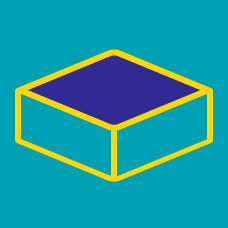Geometry

# Volume: Level 1 Challenges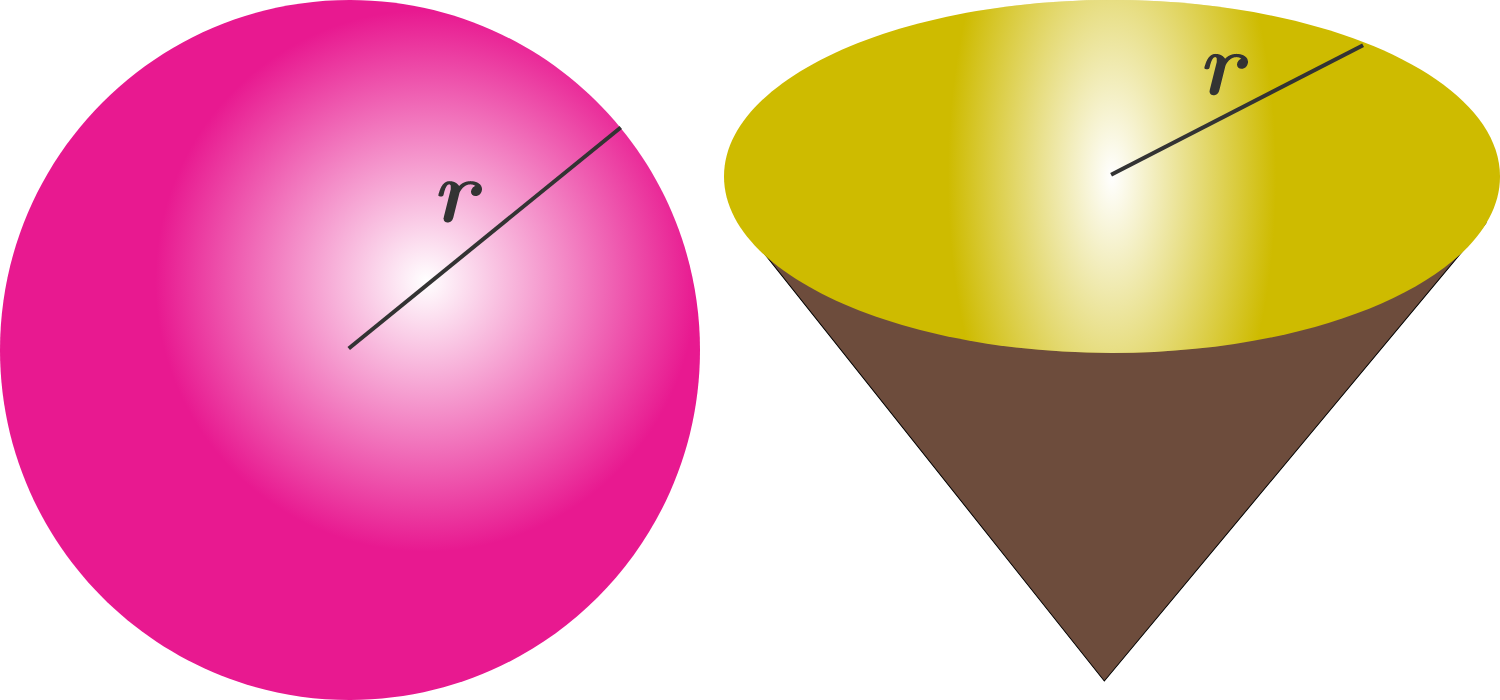A spherical scoop of ice cream has the same radius as the base of a cone. The heights of the cone and the scoop of ice cream are the same.

If the sphere of ice cream were to melt, then how many ice cream cones of this type could it fill?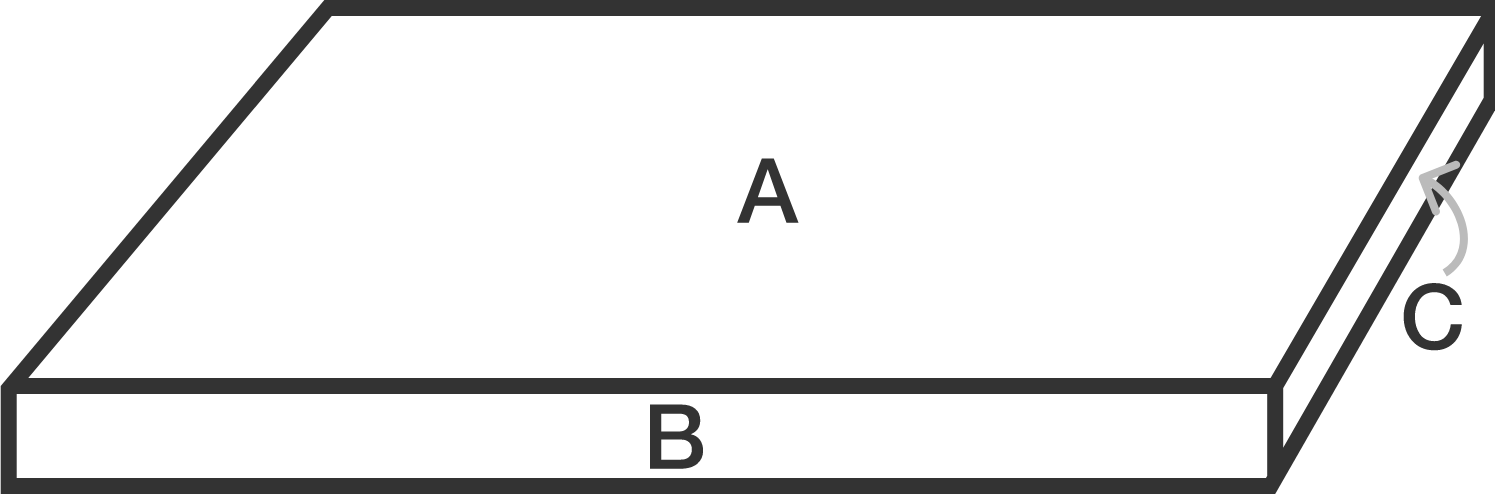The diagram above shows a rectangular prism. Given that the surface area of surface $A$, $B$, and $C$ are 1024, 81 and 49 respectively, find the volume of the cuboid.

Below shows a sphere inscribed inside a cylinder. Find the ratio of volumes between the sphere and the cylinder.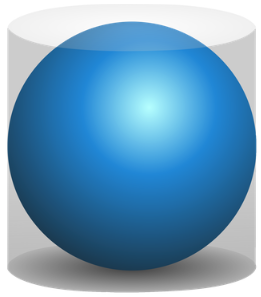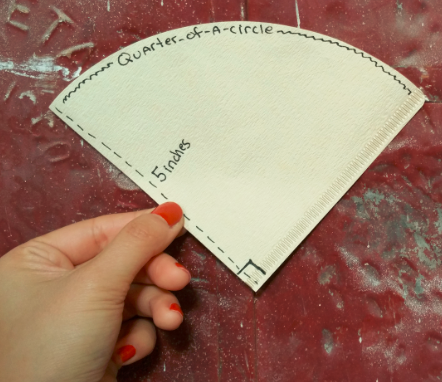When a conical coffee filter is empty, it lies flat as a two-layers-thick, quarter circle with an edge length of 5 inches. What is the height of the coffee filter when it is filled with coffee grounds?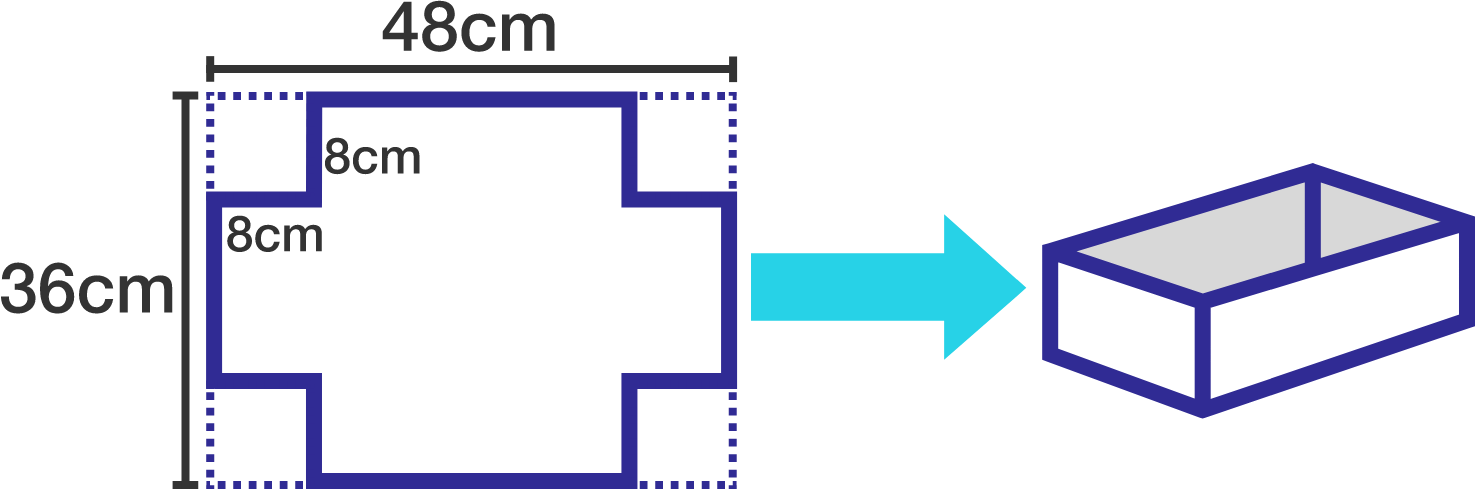A metallic sheet is of rectangular shape with dimensions $48 \text{ cm} \times 36 \text{ cm }$. From each of its corners, a square of $8 \text{ cm}$ is cut. An open box is made from the remaining part of the sheet. Find the volume of the box.

×